# Floor

The floor area of ​​the room is 31 m2 and has a width of 4.3 m. How many centimeters of circumference measured the floor on the map at the scale 1:75?

o =  30.7 cm

### Step-by-step explanation: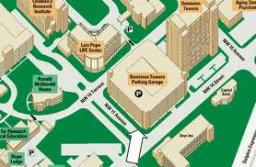Did you find an error or inaccuracy? Feel free to write us. Thank you!Tips to related online calculators
Need help to calculate sum, simplify or multiply fractions? Try our fraction calculator.
Check out our ratio calculator.
Do you want to convert length units?

#### You need to know the following knowledge to solve this word math problem:

We encourage you to watch this tutorial video on this math problem:

## Related math problems and questions:

• FloorRectangular floor of living room has a length 5.4 meters and a circumference 17.2 meters. What is its width?
• Carpet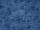The room has a rectangle shape with dimensions 5 m × 2.1 m. How many meters of carpet a width of 2 need to buy to cover the entire room?
• Map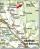Forest has an area of ​​36 ha. How much area is occupied by forest on the map at scale 1:500?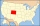The state Colorado has the shape of a rectangle. The map is sold on 2 posters. The first has dimensions of 70 cm and 50 cm in a scale of 1: 1,000,000. The second poster has dimensions of 175 cm and 125 cm. What is the scale of the second poster?
• ScaleThe swimming pool is long 110 m and 30 m wide. The plan of the city is shown as a rectangle with an area 8.25 cm2. What scale is the city plan?
• Map scale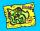What is the scale of the map, if 5.4 km is 72 mm on the map?
• Map scaleGarden has on plan on a scale of 1: 150 width 22 cm and length 35 cm. What is the real area of the garden?
• Scale 3Miriam room is 3.2 meters wide. It is draw by line segment length 6.4 cm on floor plan. In what scale it is plan of the room?
• On the floor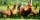You enter the room and there are 2 dogs, 4 horses, 1 giraffe and one duck on the bed, 3 chickens are flying over the chair. How many feet are on the floor?
• Map scaleThe rectangular plot has a scale of 1: 10000 area 3 cm2 on the map. What content does this plot have on a 1:5000 scale map?
• Scale of planOn the plan of the village in the scale of 1: 1000 a rectangular garden is drawn. Its dimensions on the plan are 25mm and 28mm. Determine the area of the garden in ares.
• Map scaleOn a 1:1000 scale map is a rectangular land of 4.2 cm and 5.8 cm. What is the area of this land in square meters?
• Rectangular gardenRectangular garden has a length of 48.7 m, a width of 6.3 meters shorter than the length. How much mesh should be bought for its fencing if the gate is 2.9 m long and the gate 1.1 m? What is the area of the garden?
• Room dimensions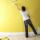Room dimensions are 5m and 3.5m. Room height is 2.85m. Paint the room (even with the ceiling). There will be 2 layers. Doors and windows have a total of 2.5 m2. One box of paint is enough for 6m2. How many boxes of paint are needed? How much do we pay if
• Floating floor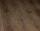In the new building is considered a floating floor worker in each room. 30 minutes lay 9 m2 of the floor. How long 3 workers laid the floor in 4 rooms with a total area of 90 m2?
• The perimeter 2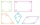The perimeter of the quadrilateral a = 1m b = 14/5m c = 2 3/10m d = 1 4/5m?
• Hectares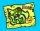Determine the area of the rectangular land (in ha), which has dimensions of 3 cm and 4.5 cm on the plan with a scale of 1: 40,000.